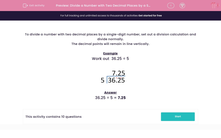# Divide a Number with Two Decimal Places by a Single-Digit Number

In this worksheet, students divide a number with two decimal places by a single-digit number.This content is premium and exclusive to EdPlace subscribers.Key stage:  KS 2

Curriculum topic:   Maths and Numerical Reasoning

Curriculum subtopic:   Decimals

Difficulty level:#### Worksheet Overview

To divide a number with two decimal places by a single-digit number, set out a division calculation and divide normally.

The decimal points will remain in line vertically.

Example

Work out  36.25 ÷ 5